Courses

# Test: Electromagnetic Field Theory - 3

## 25 Questions MCQ Test Electromagnetic Theory | Test: Electromagnetic Field Theory - 3

Description
This mock test of Test: Electromagnetic Field Theory - 3 for Electrical Engineering (EE) helps you for every Electrical Engineering (EE) entrance exam. This contains 25 Multiple Choice Questions for Electrical Engineering (EE) Test: Electromagnetic Field Theory - 3 (mcq) to study with solutions a complete question bank. The solved questions answers in this Test: Electromagnetic Field Theory - 3 quiz give you a good mix of easy questions and tough questions. Electrical Engineering (EE) students definitely take this Test: Electromagnetic Field Theory - 3 exercise for a better result in the exam. You can find other Test: Electromagnetic Field Theory - 3 extra questions, long questions & short questions for Electrical Engineering (EE) on EduRev as well by searching above.
QUESTION: 1

### A material has conductivity of 10-2 mho/m and a relative permittivity of 4. The frequency at which conduction current in the medium is equal to displacement current is

Solution:

Conduction current density J = wD

J = σE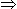σE = ωD = 2p fD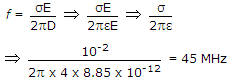QUESTION: 2

### A transmission line is feeding 1 watt of power to a horn antenna having a gain of 10 dB. The antenna is matched to the transmission line. The total power radiated by the horn antenna into the free space is

Solution:

Pradiated = Gain of Antenna x Input power = G.I10 log1010 . 110 watt.

QUESTION: 3

### An end fire array consisting of several half-wave lengths of directive gain 40, then find the width of major lobe

Solution: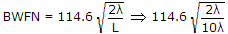.

QUESTION: 4

Poynting vector is associated with which of the following?

Solution:

P = E x H

QUESTION: 5

A uniform plane EM wave incident normally on a plane surface of a dielectric material is reflected with a VSWR of 3. What percentage of incident power that is reflected?

Solution: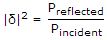Preflected = |δ|2 Pincident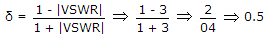|δ|2 = 0.25 .

QUESTION: 6

A 10 GHz signal propagates in a waveguide with a phase velocity of 4 x 108 m/s. If the signal produces standing wave in the guide, then the distance between successive minimal will be

Solution:

f = 10 GHz, v = 4 x 108 m/sec,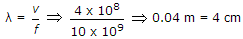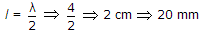.

QUESTION: 7

The incidence from dielectric medium 1 (ε1) into dielectric medium 2 (ε2), Brewster angle θB and the corresponding angle of transmission θt for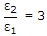will be respectively

Solution:

Brewster angle θ = tan-1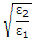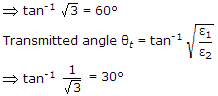QUESTION: 8

A medium of relative permittivity εr2 = 2 forms an interface with free space. A point source of EM energy is located in the medium at a depth of 1 meter from the interface. Due to the total internal reflection, the transmitted beam has a circular cross-section over the interface. The area of the beam cross section at the interface is given by

Solution: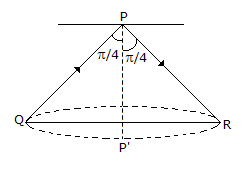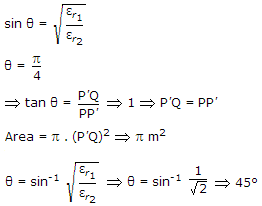QUESTION: 9

A transmission line of characteristic impedance z0 = 50 Ω. Phase Velocity = 2 x 108 m/sec, and length l = 1 m is terminated by a load z1 = (30 - j 40) ohms. The input impedance of the line for a frequency of 100 MHz will be

Solution: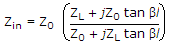.

QUESTION: 10

A circular waveguide carries TE11 mode whose radial electric-field is given by
Er = E 0J1 (r) sin φ V/m
where 'r' is the radial distance in cm, from the waveguide axis. The cut-off wavelength of the mode is

Solution: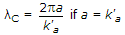.

QUESTION: 11

A uniform plane wave travelling in air is incident on the plane boundary between air and another dielectric medium with εr = 4. The reflection coefficient for the normal incidence is

Solution:

Reflection coefficient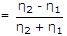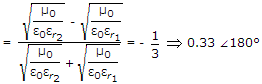.

QUESTION: 12

A transmission line has characteristics impedance of (75 + j 0.01) Ω and is terminated in a load impedance of (70 + j 50) Ω. The transmission coefficient will be

Solution:

Transmission coefficient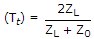.

QUESTION: 13

Maximum effective aperture of an antenna which is operating at a wavelength of 3 meters and has a directivity of 100

Solution: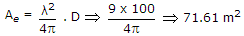.

QUESTION: 14

The distance between maxima and minima of a standing wave is

Solution: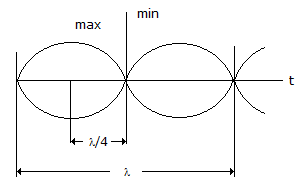QUESTION: 15

A TV. transmitting aerial is fixed on top of a 150 metre tower located on a mountain 1200 m height. The range of transmitter is

Solution:

1 = 1.44[ht + ht]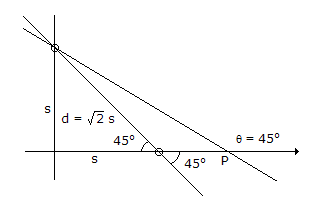QUESTION: 16

A vertical wire of 1 meter length carries a current of 1 A at 10 MHz. The total radiated power is nearly

Solution:

P = I2 . R(1)2 . 7373 watt.

Here, vertical wire will be considered as dipole.

QUESTION: 17

The length of half-wave antenna is at 30 MHz

Solution: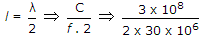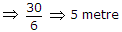.

QUESTION: 18

For a 400 kHz transmission line having L = 0.5 mH/km, C = 0.08 mF and negligible R and G, the characteristic impedance will be

Solution:

f = 400 kHz, L = 0.5 mH, C = 0.08 mF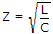.

QUESTION: 19

Which one of the following statement is not a correct for a plane wave with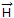= 0.5e-0.1x cos (106t - 2x)az A/m?

Solution:

P = E x HP = EH, θ = 90º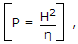η = 120p = 377 Ω.

QUESTION: 20

Beamwidth (between first nulls) will be for an antenna at 3 MHz with directivity of 100

Solution: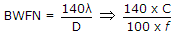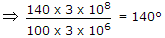.

QUESTION: 21

If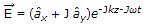and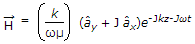the time average Poynting vector is

Solution: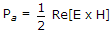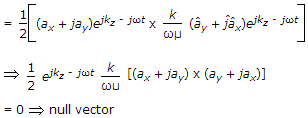QUESTION: 22

Calculate the wave number in waveguide having dimensions 4 x 2 cm at 3 GHz

Solution: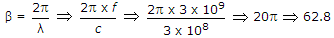.

QUESTION: 23

For a transmission line with homogeneous dielectric the capacitance per unit length is 'c', the relative permittivity of the dielectric is 'εr' and velocity of light in free space is 'v' the characteristic impedance z0 is equal to

Solution: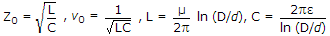.

QUESTION: 24

The I/P impedance of a short circuited quarter wave long transmission line is

Solution:

Because for irrotational, ∇ x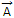= 0.

QUESTION: 25

A dipole antenna of λ/8 length has an equivalent total loss resistance of 1.5 Ω. The efficiency of the antenna is

Solution:

Rr = 80p2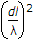, Re = 1.5 Ω,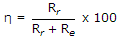.